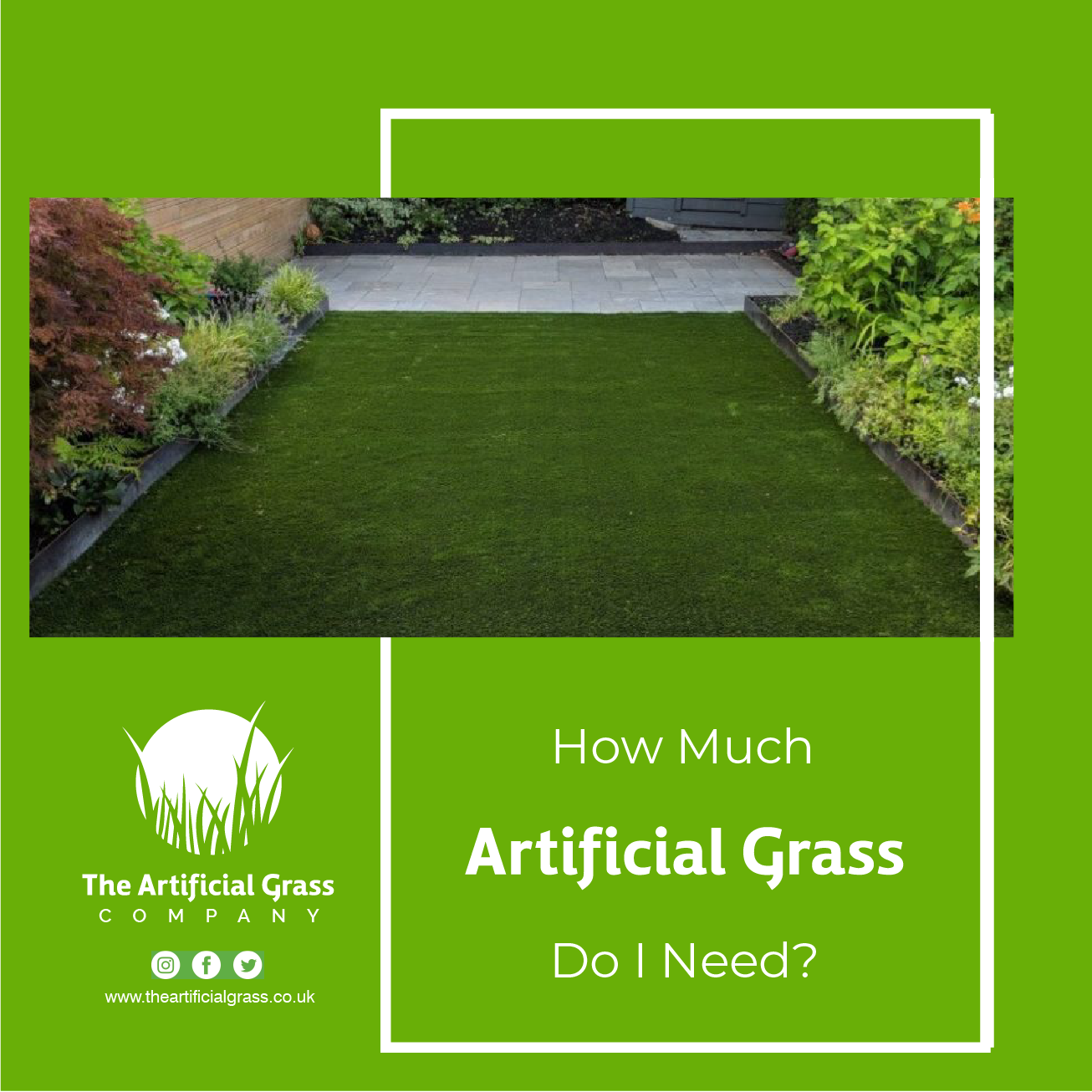#### How Much Artificial Grass Do I Need?

If you are interested in having artificial grass but are confused, How Much Artificial Grass Do I Need? The answer depends on how big the lawn or installation area is. The more extensive area, the more materials, time, and labour are needed, so that you will need more artificial grass. You're seriously considering artificial grass for your Lawn. You want to answer the question, "How Much Artificial Grass Do I Need" for My Lawn?" The first step is to measure the area in square metres of your Lawn. That isn't too confusing, but you'll need a few simple steps.

Follow us on Instagram; The Artificial Grass Company UK

#### How Much Artificial Grass Do I Need?

If you have a rectangle or square lawn, congratulations! You are the lucky ones, and measuring it is pretty simple. You will have to calculate the length and width of the rectangle or square in metre to calculate the amount of artificial grass required. Once you know the length and width, multiply it. For example,

6 m in length and 5 m in width.

6m x 5m = 30m

##### How Much Artificial Grass Do I Need for the circle lawn?

The most innovative method to get an idea of how much artificial grass you will need to cover a circular lawn is to calculate the diameter of the area in metres. Once the diameter is identified, multiply with 0.80, then times it by the diameter again. For example:

Diameter = 12m

12m x 0.80 = 9.6m

9.6x 12m = 115.2m²

##### How Much Artificial Grass Do I Need for the triangle lawn?

To work out how much artificial grass you will need to cover a triangle-shaped lawn, measure the base and height of the area in metres, and then multiply the base by 0.50 before multiplying that by the height, for example.

Base = 17m. Height = 13m.

17 x 0.50 x 13 = 110.5 m²

##### How Much Artificial Grass Do I Need for an oval lawn?

To calculate how much artificial grass you will need to protect an oval lawn, measure the length and width in metres, then multiply the length by 0.80 before multiplying it by the width. For example,

8 m in length, 12 m in width.

8m x 0.80 x 12m = 76.8m²

Also Read: Will Artificial Grass Rot my Decking?